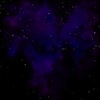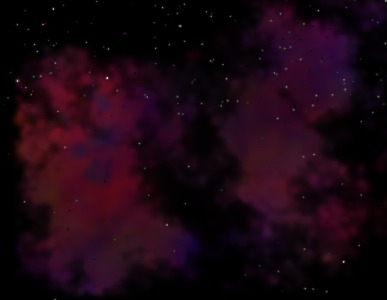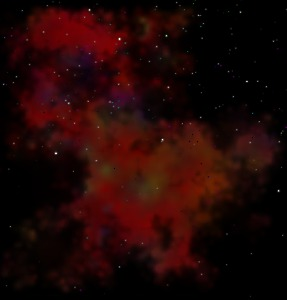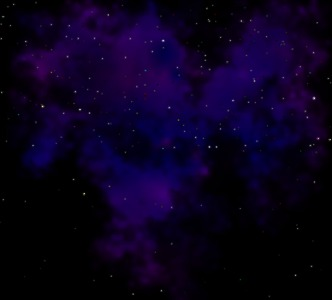# The Developer’s Cry

Yet another blog by a hobbyist programmer

# Rendering random nebulae (part 2)Last time, I talked about how to render a random nebula. As a final remark, I stated that the nebula had no shape; the plasma simply fills the entire image to its boundaries. The result is that you end up with a texture that is unusable; you can not have a nebula in the starry sky that is shaped perfectly like a square. So, we need to give it a random shape.

I tried fading out at the edges, and I tried a simple circular fade. It doesn’t work. In the first case, you end up with a perfect square, that perfectly fades out to the edges. In the second case, you end up with a perfect circle. Both look like crap, we really need a random shape.

I decided the alpha channel of the nebula should be like a height map, with height zero around the edges. I tried out the diamond-square algorithm, which seems ideally suited for this (by the way, the plasma of the nebula is generated using a diamond-square algorithm), but it’s hard to control around the edges. I thought about leveling the edges by ‘pushing a pyramid’ down over the image, but I’m sure it wouldn’t look nice.

How do you generate random shapes? The answer: with a fractal. The word ‘fractal’ sounds very mathematical and difficult, but its not. See wikipedia for some info.
Algorithm used to generate a fractal shape:

1. draw a circle;
2. choose a random point on the edge of the circle;
3. divide the radius by two;
4. recurse; draw smaller circle at the newly chosen point.

Using a circle as a brush is not a bad choice, but if the edges have to fade, then the brush should be a radial gradient. I had a little trouble in drawing a radial gradient and did not find the right solution online, so I’ll share my formula with you. It’s actually easier than you might think:

``````float d = sqrt(dx * dx + dy * dy);
``````

`d` is the distance of the pixel to the center of the circle.
`gradient` is the difference between the low end and the high end of the gradient; usually 255 for a full spectrum.

I work with overlapping circles in the alpha channel, so I use:

``````new_alpha = old_alpha + the gradient formula
``````

When you do this, you will get a lot of high values in the alpha channel as they add up quickly, and that doesn’t look good for nebulae. Therefore I chose a small value for the gradient variable, and then the results get quite nice.
Here are some small screenshots: